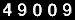### Felix Abramovich

#### Tel Aviv University

Ramat Aviv, Tel Aviv 69978
Israel
+972-3-6405389

#### Book##### Statistical Theory: A Concise Introduction
Abramovich, F. and Ritov, Y. (2013).
Chapman & Hall/CRC
Summary: Designed for a one-semester advanced undergraduate or graduate course, Statistical Theory: A Concise Introduction clearly explains the underlying ideas and principles of major statistical concepts, including parameter estimation, confidence intervals, hypothesis testing, asymptotic analysis, Bayesian inference, and elements of decision theory. It introduces these topics on a clear intuitive level using illustrative examples in addition to the formal definitions, theorems, and proofs. Based on the authors' lecture notes, this student-oriented, self-contained book maintains a proper balance between the clarity and rigor of exposition. In a few cases, the authors present a "sketched" version of a proof, explaining its main ideas rather than giving detailed technical mathematical and probabilistic arguments. Chapters and sections marked by asterisks contain more advanced topics and may be omitted. A special chapter on linear models shows how the main theoretical concepts can be applied to the well-known and frequently used statistical tool of linear regression. Requiring no heavy calculus, simple questions throughout the text help students check their understanding of the material. Each chapter also includes a set of exercises that range in level of difficulty.

#### Research Interests:

• statistical learning
• high-dimensional inference, sparsity, model selection procedures
• nonparametric curve estimation and related problems
• wavelets in statistics

#### Professional Associations:

According to WEB counter you are Visitor No: